### Home > A2C > Chapter 10 > Lesson 10.2.2 > Problem10-78

10-78.
1. Write an equivalent expression for each of the following situations that does not include the factorial (!) symbol. Homework Help ✎

1. The first five factors of (n − 3)!

2. The first five factors of (n + 2)!

3.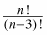4.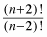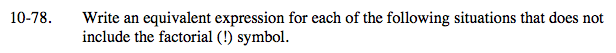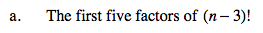(n − 3) (n − 4) (n − 5) (n − 6) (n − 7)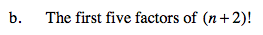See part (a).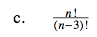$\frac{n!}{(n-3)!}=\frac{n(n-1)(n-2)(n-3)(n-4)...}{(n-3)(n-4)...}$

n(n − 1) (n − 2)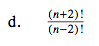See part (c).